Oscillations

Pendulums

Learning Objectives

By the end of this section, you will be able to:

• State the forces that act on a simple pendulum
• Determine the angular frequency, frequency, and period of a simple pendulum in terms of the length of the pendulum and the acceleration due to gravity
• Define the period for a physical pendulum
• Define the period for a torsional pendulum

Pendulums are in common usage. Grandfather clocks use a pendulum to keep time and a pendulum can be used to measure the acceleration due to gravity. For small displacements, a pendulum is a simple harmonic oscillator.

The Simple Pendulum

A simple pendulum is defined to have a point mass, also known as the pendulum bob, which is suspended from a string of length L with negligible mass ((Figure)). Here, the only forces acting on the bob are the force of gravity (i.e., the weight of the bob) and tension from the string. The mass of the string is assumed to be negligible as compared to the mass of the bob.

A simple pendulum has a small-diameter bob and a string that has a very small mass but is strong enough not to stretch appreciably. The linear displacement from equilibrium is s, the length of the arc. Also shown are the forces on the bob, which result in a net force of $\text{−}mg\text{sin}\phantom{\rule{0.2em}{0ex}}\theta$ toward the equilibrium position—that is, a restoring force.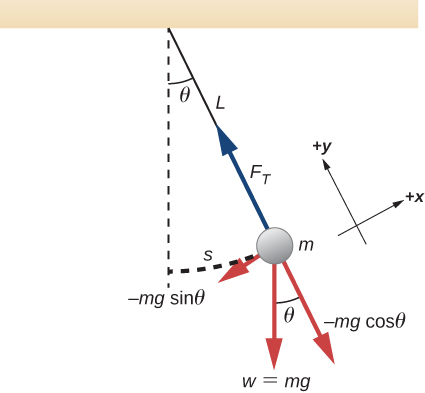Consider the torque on the pendulum. The force providing the restoring torque is the component of the weight of the pendulum bob that acts along the arc length. The torque is the length of the string L times the component of the net force that is perpendicular to the radius of the arc. The minus sign indicates the torque acts in the opposite direction of the angular displacement:

$\begin{array}{ccc}\hfill \tau & =\hfill & \text{−}L\left(mg\phantom{\rule{0.2em}{0ex}}\text{sin}\phantom{\rule{0.2em}{0ex}}\theta \right);\hfill \\ \hfill I\alpha & =\hfill & \text{−}L\left(mg\phantom{\rule{0.2em}{0ex}}\text{sin}\phantom{\rule{0.2em}{0ex}}\theta \right);\hfill \\ \hfill I\frac{{d}^{2}\theta }{d{t}^{2}}& =\hfill & \text{−}L\left(mg\phantom{\rule{0.2em}{0ex}}\text{sin}\phantom{\rule{0.2em}{0ex}}\theta \right);\hfill \\ \hfill m{L}^{2}\frac{{d}^{2}\theta }{d{t}^{2}}& =\hfill & \text{−}L\left(mg\phantom{\rule{0.2em}{0ex}}\text{sin}\phantom{\rule{0.2em}{0ex}}\theta \right);\hfill \\ \hfill \frac{{d}^{2}\theta }{d{t}^{2}}& =\hfill & -\frac{g}{L}\text{sin}\phantom{\rule{0.2em}{0ex}}\theta .\hfill \end{array}$

The solution to this differential equation involves advanced calculus, and is beyond the scope of this text. But note that for small angles (less than 15 degrees), $\text{sin}\phantom{\rule{0.2em}{0ex}}\theta$ and $\theta$ differ by less than 1%, so we can use the small angle approximation $\text{sin}\phantom{\rule{0.2em}{0ex}}\theta \approx \theta .$ The angle $\theta$ describes the position of the pendulum. Using the small angle approximation gives an approximate solution for small angles,

$\frac{{d}^{2}\theta }{d{t}^{2}}=-\frac{g}{L}\theta .$

Because this equation has the same form as the equation for SHM, the solution is easy to find. The angular frequency is

$\omega =\sqrt{\frac{g}{L}}$

and the period is

$T=2\pi \sqrt{\frac{L}{g}}.$

The period of a simple pendulum depends on its length and the acceleration due to gravity. The period is completely independent of other factors, such as mass and the maximum displacement. As with simple harmonic oscillators, the period T for a pendulum is nearly independent of amplitude, especially if $\theta$ is less than about $15\text{°}.$ Even simple pendulum clocks can be finely adjusted and remain accurate.

Note the dependence of T on g. If the length of a pendulum is precisely known, it can actually be used to measure the acceleration due to gravity, as in the following example.

Measuring Acceleration due to Gravity by the Period of a Pendulum
What is the acceleration due to gravity in a region where a simple pendulum having a length 75.000 cm has a period of 1.7357 s?

Strategy
We are asked to find g given the period T and the length L of a pendulum. We can solve $T=2\pi \sqrt{\frac{L}{g}}$ for g, assuming only that the angle of deflection is less than $15\text{°}$.

Solution

1. Square $T=2\pi \sqrt{\frac{L}{g}}$ and solve for g:
$g=4{\pi }^{2}\frac{L}{{T}^{2}}.$
2. Substitute known values into the new equation:
$g=4{\pi }^{2}\frac{0.75000\phantom{\rule{0.2em}{0ex}}\text{m}}{{\left(1.7357\phantom{\rule{0.2em}{0ex}}\text{s}\right)}^{2}}.$
3. Calculate to find g:
$g=9.8281{\phantom{\rule{0.2em}{0ex}}\text{m/s}}^{2}.$

Significance
This method for determining g can be very accurate, which is why length and period are given to five digits in this example. For the precision of the approximation $\text{sin}\phantom{\rule{0.2em}{0ex}}\theta \approx \theta$ to be better than the precision of the pendulum length and period, the maximum displacement angle should be kept below about $0.5\text{°}$.

Check Your Understanding An engineer builds two simple pendulums. Both are suspended from small wires secured to the ceiling of a room. Each pendulum hovers 2 cm above the floor. Pendulum 1 has a bob with a mass of 10 kg. Pendulum 2 has a bob with a mass of 100 kg. Describe how the motion of the pendulums will differ if the bobs are both displaced by $12\text{°}$.

The movement of the pendulums will not differ at all because the mass of the bob has no effect on the motion of a simple pendulum. The pendulums are only affected by the period (which is related to the pendulum’s length) and by the acceleration due to gravity.

Physical Pendulum

Any object can oscillate like a pendulum. Consider a coffee mug hanging on a hook in the pantry. If the mug gets knocked, it oscillates back and forth like a pendulum until the oscillations die out. We have described a simple pendulum as a point mass and a string. A physical pendulum is any object whose oscillations are similar to those of the simple pendulum, but cannot be modeled as a point mass on a string, and the mass distribution must be included into the equation of motion.

As for the simple pendulum, the restoring force of the physical pendulum is the force of gravity. With the simple pendulum, the force of gravity acts on the center of the pendulum bob. In the case of the physical pendulum, the force of gravity acts on the center of mass (CM) of an object. The object oscillates about a point O. Consider an object of a generic shape as shown in (Figure).

A physical pendulum is any object that oscillates as a pendulum, but cannot be modeled as a point mass on a string. The force of gravity acts on the center of mass (CM) and provides the restoring force that causes the object to oscillate. The minus sign on the component of the weight that provides the restoring force is present because the force acts in the opposite direction of the increasing angle $\theta$.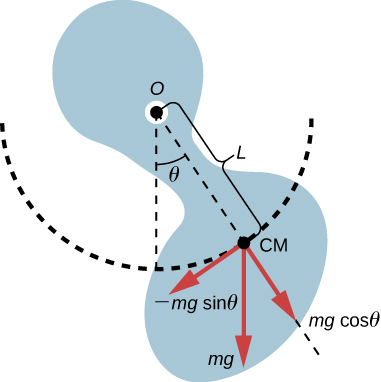When a physical pendulum is hanging from a point but is free to rotate, it rotates because of the torque applied at the CM, produced by the component of the object’s weight that acts tangent to the motion of the CM. Taking the counterclockwise direction to be positive, the component of the gravitational force that acts tangent to the motion is $\text{−}mg\phantom{\rule{0.2em}{0ex}}\text{sin}\phantom{\rule{0.2em}{0ex}}\theta$. The minus sign is the result of the restoring force acting in the opposite direction of the increasing angle. Recall that the torque is equal to $\stackrel{\to }{\tau }=\stackrel{\to }{r}\phantom{\rule{0.2em}{0ex}}×\phantom{\rule{0.2em}{0ex}}\stackrel{\to }{F}$. The magnitude of the torque is equal to the length of the radius arm times the tangential component of the force applied, $|\tau |=rF\text{sin}\phantom{\rule{0.2em}{0ex}}\theta$. Here, the length L of the radius arm is the distance between the point of rotation and the CM. To analyze the motion, start with the net torque. Like the simple pendulum, consider only small angles so that $\text{sin}\phantom{\rule{0.2em}{0ex}}\theta \approx \theta$. Recall from Fixed-Axis Rotation on rotation that the net torque is equal to the moment of inertia $I=\int {r}^{2}dm$ times the angular acceleration $\alpha ,$ where $\alpha =\frac{{d}^{2}\theta }{d{t}^{2}}$:

$I\alpha ={\tau }_{\text{net}}=L\left(\text{−}mg\right)\text{sin}\phantom{\rule{0.2em}{0ex}}\theta .$

Using the small angle approximation and rearranging:

$\begin{array}{ccc}\hfill I\alpha & =\hfill & \text{−}L\left(mg\right)\theta ;\hfill \\ \hfill I\frac{{d}^{2}\theta }{d{t}^{2}}& =\hfill & \text{−}L\left(mg\right)\theta ;\hfill \\ \hfill \frac{{d}^{2}\theta }{d{t}^{2}}& =\hfill & \text{−}\left(\frac{mgL}{I}\right)\theta .\hfill \end{array}$

Once again, the equation says that the second time derivative of the position (in this case, the angle) equals minus a constant $\left(-\frac{mgL}{I}\right)$ times the position. The solution is

$\theta \left(t\right)=\text{Θ}\text{cos}\left(\omega t+\varphi \right),$

where $\text{Θ}$ is the maximum angular displacement. The angular frequency is

$\omega =\sqrt{\frac{mgL}{I}}.$

The period is therefore

$T=2\pi \sqrt{\frac{I}{mgL}}.$

Note that for a simple pendulum, the moment of inertia is $I=\int {r}^{2}dm=m{L}^{2}$ and the period reduces to $T=2\pi \sqrt{\frac{L}{g}}$.

Reducing the Swaying of a Skyscraper
In extreme conditions, skyscrapers can sway up to two meters with a frequency of up to 20.00 Hz due to high winds or seismic activity. Several companies have developed physical pendulums that are placed on the top of the skyscrapers. As the skyscraper sways to the right, the pendulum swings to the left, reducing the sway. Assuming the oscillations have a frequency of 0.50 Hz, design a pendulum that consists of a long beam, of constant density, with a mass of 100 metric tons and a pivot point at one end of the beam. What should be the length of the beam?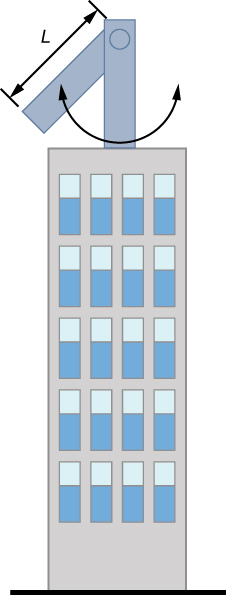Strategy
We are asked to find the length of the physical pendulum with a known mass. We first need to find the moment of inertia of the beam. We can then use the equation for the period of a physical pendulum to find the length.

Solution

1. Find the moment of inertia for the CM:
2. Use the parallel axis theorem to find the moment of inertia about the point of rotation:
$I={I}_{\text{CM}}+{\frac{L}{4}}^{2}M=\frac{1}{12}M{L}^{2}+\frac{1}{4}M{L}^{2}=\frac{1}{3}M{L}^{2}.$
3. The period of a physical pendulum has a period of $T=2\pi \sqrt{\frac{I}{mgL}}$. Use the moment of inertia to solve for the length L:
$\begin{array}{ccc}\hfill T& =\hfill & 2\pi \sqrt{\frac{I}{MgL}}=2\pi \sqrt{\frac{\frac{1}{3}M{L}^{2}}{MgL}}=2\pi \sqrt{\frac{L}{3g}};\hfill \\ \hfill L& =\hfill & 3g{\left(\frac{T}{2\pi }\right)}^{2}=3\left(9.8\frac{\text{m}}{{\text{s}}^{2}}\right){\left(\frac{2\phantom{\rule{0.2em}{0ex}}\text{s}}{2\pi }\right)}^{2}=2.98\phantom{\rule{0.2em}{0ex}}\text{m}\text{.}\hfill \end{array}$

Significance
There are many ways to reduce the oscillations, including modifying the shape of the skyscrapers, using multiple physical pendulums, and using tuned-mass dampers.

Torsional Pendulum

A torsional pendulum consists of a rigid body suspended by a light wire or spring ((Figure)). When the body is twisted some small maximum angle $\left(\text{Θ}\right)$ and released from rest, the body oscillates between $\left(\theta =+\text{Θ}\right)$ and $\left(\theta =\text{−}\phantom{\rule{0.2em}{0ex}}\text{Θ}\right)$. The restoring torque is supplied by the shearing of the string or wire.

A torsional pendulum consists of a rigid body suspended by a string or wire. The rigid body oscillates between $\theta =+\text{Θ}$ and $\theta =\text{−}\text{Θ}$.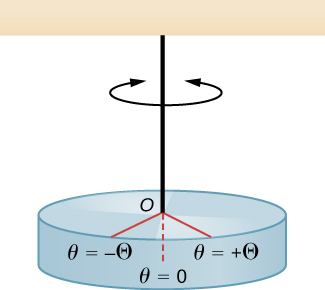The restoring torque can be modeled as being proportional to the angle:

$\tau =\text{−}\kappa \theta .$

The variable kappa $\left(\kappa \right)$ is known as the torsion constant of the wire or string. The minus sign shows that the restoring torque acts in the opposite direction to increasing angular displacement. The net torque is equal to the moment of inertia times the angular acceleration:

$\begin{array}{}\\ I\frac{{d}^{2}\theta }{d{t}^{2}}=\text{−}\kappa \theta ;\hfill \\ \frac{{d}^{2}\theta }{d{t}^{2}}=-\frac{\kappa }{I}\theta .\hfill \end{array}$

This equation says that the second time derivative of the position (in this case, the angle) equals a negative constant times the position. This looks very similar to the equation of motion for the SHM $\frac{{d}^{2}x}{d{t}^{2}}=-\frac{k}{m}x$, where the period was found to be $T=2\pi \sqrt{\frac{m}{k}}$. Therefore, the period of the torsional pendulum can be found using

$T=2\pi \sqrt{\frac{I}{\kappa }}.$

The units for the torsion constant are $\left[\kappa \right]=\text{N-m}=\left(\text{kg}\frac{\text{m}}{{\text{s}}^{2}}\right)\text{m}=\text{kg}\phantom{\rule{0.2em}{0ex}}\frac{{\text{m}}^{2}}{{\text{s}}^{2}}$ and the units for the moment of inertial are $\left[I\right]={\text{kg-m}}^{2},$ which show that the unit for the period is the second.

Measuring the Torsion Constant of a String
A rod has a length of $l=0.30\phantom{\rule{0.2em}{0ex}}\text{m}$ and a mass of 4.00 kg. A string is attached to the CM of the rod and the system is hung from the ceiling ((Figure)). The rod is displaced 10 degrees from the equilibrium position and released from rest. The rod oscillates with a period of 0.5 s. What is the torsion constant $\kappa$?

(a) A rod suspended by a string from the ceiling. (b) Finding the rod’s moment of inertia.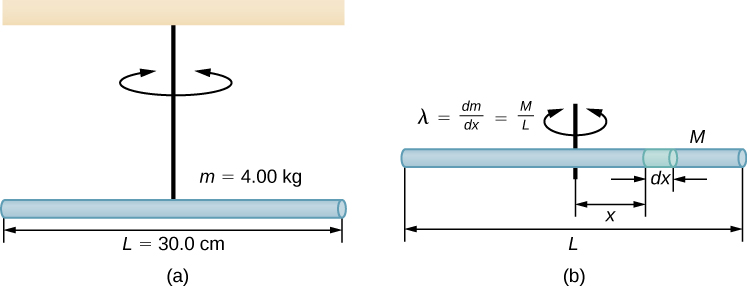Strategy
We are asked to find the torsion constant of the string. We first need to find the moment of inertia.

Solution

1. Find the moment of inertia for the CM:
${I}_{\text{CM}}=\int {x}^{2}dm={\int }_{\text{−}L\text{/}2}^{+L\text{/}2}{x}^{2}\lambda dx=\lambda {\left[\frac{{x}^{3}}{3}\right]}_{\text{−}L\text{/}2}^{+L\text{/}2}=\lambda \frac{2{L}^{3}}{24}=\left(\frac{M}{L}\right)\frac{2{L}^{3}}{24}=\frac{1}{12}M{L}^{2}.$
2. Calculate the torsion constant using the equation for the period:
$\begin{array}{ccc}\hfill T& =\hfill & 2\pi \sqrt{\frac{I}{\kappa }};\hfill \\ \hfill \kappa & =\hfill & I{\left(\frac{2\pi }{T}\right)}^{2}=\left(\frac{1}{12}M{L}^{2}\right){\left(\frac{2\pi }{T}\right)}^{2};\hfill \\ & =\hfill & \left(\frac{1}{12}\left(4.00\phantom{\rule{0.2em}{0ex}}\text{kg}\right){\left(0.30\phantom{\rule{0.2em}{0ex}}\text{m}\right)}^{2}\right){\left(\frac{2\pi }{0.50\phantom{\rule{0.2em}{0ex}}\text{s}}\right)}^{2}=4.73\phantom{\rule{0.2em}{0ex}}\text{N}·\text{m}\text{.}\hfill \end{array}$

Significance
Like the force constant of the system of a block and a spring, the larger the torsion constant, the shorter the period.

Summary

• A mass m suspended by a wire of length L and negligible mass is a simple pendulum and undergoes SHM for amplitudes less than about $15\text{°}$. The period of a simple pendulum is $T=2\pi \sqrt{\frac{L}{g}}$, where L is the length of the string and g is the acceleration due to gravity.
• The period of a physical pendulum $T=2\pi \sqrt{\frac{I}{mgL}}$ can be found if the moment of inertia is known. The length between the point of rotation and the center of mass is L.
• The period of a torsional pendulum $T=2\pi \sqrt{\frac{I}{\kappa }}$ can be found if the moment of inertia and torsion constant are known.

Conceptual Questions

Pendulum clocks are made to run at the correct rate by adjusting the pendulum’s length. Suppose you move from one city to another where the acceleration due to gravity is slightly greater, taking your pendulum clock with you, will you have to lengthen or shorten the pendulum to keep the correct time, other factors remaining constant? Explain your answer.

A pendulum clock works by measuring the period of a pendulum. In the springtime the clock runs with perfect time, but in the summer and winter the length of the pendulum changes. When most materials are heated, they expand. Does the clock run too fast or too slow in the summer? What about the winter?

The period of the pendulum is $T=2\pi \sqrt{L\text{/}g}.$ In summer, the length increases, and the period increases. If the period should be one second, but period is longer than one second in the summer, it will oscillate fewer than 60 times a minute and clock will run slow. In the winter it will run fast.

With the use of a phase shift, the position of an object may be modeled as a cosine or sine function. If given the option, which function would you choose? Assuming that the phase shift is zero, what are the initial conditions of function; that is, the initial position, velocity, and acceleration, when using a sine function? How about when a cosine function is used?

Problems

What is the length of a pendulum that has a period of 0.500 s?

Some people think a pendulum with a period of 1.00 s can be driven with “mental energy” or psycho kinetically, because its period is the same as an average heartbeat. True or not, what is the length of such a pendulum?

24.8 cm

What is the period of a 1.00-m-long pendulum?

How long does it take a child on a swing to complete one swing if her center of gravity is 4.00 m below the pivot?

4.01 s

The pendulum on a cuckoo clock is 5.00-cm long. What is its frequency?

Two parakeets sit on a swing with their combined CMs 10.0 cm below the pivot. At what frequency do they swing?

1.58 s

(a) A pendulum that has a period of 3.00000 s and that is located where the acceleration due to gravity is $9.79\phantom{\rule{0.2em}{0ex}}{\text{m/s}}^{2}$ is moved to a location where the acceleration due to gravity is $9.82\phantom{\rule{0.2em}{0ex}}{\text{m/s}}^{2}$. What is its new period? (b) Explain why so many digits are needed in the value for the period, based on the relation between the period and the acceleration due to gravity.

A pendulum with a period of 2.00000 s in one location ($g=9.80{\text{m/s}}^{2}$) is moved to a new location where the period is now 1.99796 s. What is the acceleration due to gravity at its new location?

$9.82002\phantom{\rule{0.2em}{0ex}}{\text{m/s}}^{2}$

(a) What is the effect on the period of a pendulum if you double its length? (b) What is the effect on the period of a pendulum if you decrease its length by 5.00%?

Glossary

physical pendulum
any extended object that swings like a pendulum
simple pendulum
point mass, called a pendulum bob, attached to a near massless string
torsional pendulum
any suspended object that oscillates by twisting its suspension